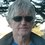# Entropy: Going From Statistical to Classical

In statistical mechanics, entropy (as per Boltzmann) is defined to be, in dimensionless form

$S=Log\left( W \right)$

where $W$ is the number of accessible microstates. For a complex system composed of subsystems $A, B, C…$ in interaction with each other, we define the total entropy as

$S=Log\left( { W }_{ A }{ W }_{ B }{ W }_{ C }... \right)$

A subsystem can be the phase space of an independent variable, as for example position or velocity. It doesn't necessarily refer to physical systems like bottles of gas that are in contact. So, for example, for an ideal gas that is composed of independently moving point-like atoms, one phase space plots all the current positions of the individual atoms while another phase space plots all the current velocities of the individual atoms.

One property of entropy is that if there are any changes among the subsystems, then the total entropy will always rise, and reach a maximum when equilibrium is reached. This is known as the 2nd Law of Thermodynamics [which is yet to be rigorously proven]. This property, in statistical mechanics, is based a number of assumptions, some of which are

1) $W$ is the number of accessible microstates, each of which has [at least roughly] equal probability of happening, or accessed

2) Each subsystem is circulating freely within their phase space of microstates

3) There is some conserved quantity or constraint or interdependence between the subsystems so that there isn’t runaway expansion in the number of microstates, if there is to be a state of equilibrium for which entropy is at maximum

4) Probability favors a subsystem being in a larger phase space than smaller

The simplest example of such a system is where

${ W }_{ A }+{ W }_{ B }=4$

so that total entropy would have a maximum at

$S=Log\left( 2\cdot 2 \right)$

For an ideal $3D$ thermodynamic gas, we assume that the governing variables are

$m, v, L, N$ or mass, root-mean-velocity, length, and number

For two subsystems of phase volumes ${ v }^{ 3N }$ and ${ L }^{ 3N }$ and for some dimensional proportionality constant $k$ we have (with $m$ having a phase space size of $1$ because it remains constant)

$S=kLog\left( W \right) =kLog\left( { v }^{ 3N }{ L }^{ 3N } \right)$

Defining physical volume to be $V={ L }^{ 3 }$, we have

$S=3NkLog\left( v\cdot { V }^{ \frac { 1 }{ 3 } } \right)$

$dS=3Nk\left( \dfrac { dv }{ v } +\dfrac { 1 }{ 3 } \dfrac { dV }{ V } \right)$

$dS=\left( Nmvdv+\dfrac { 1 }{ 3 } Nm{ v }^{ 2 }\dfrac { dV }{ V } \right) { \left( \dfrac { 1 }{ 3k } m{ v }^{ 2 } \right) }^{ -1 }$

From statistical mechanics of an ideal gas, we make use of the following definitions and relations

$U=\dfrac { 1 }{ 2 } Nm{ v }^{ 2 }$

$dU=Nm{ v }dv$

$pV=\dfrac { 1 }{ 3 } Nm{ v }^{ 2 }$

$pdV=\dfrac { 1 }{ 3 } Nm{ v }^{ 2 }\dfrac { dV }{ V }$

$\dfrac { 3 }{ 2 } NkT=U$

$T=\dfrac { 1 }{ 3k } m{ v }^{ 2 }$

where $T,p$ is temperature and pressure, and $U$ is internal energy

From these, and the expression for $dS$, we have

$dS=\dfrac { dU+pdV }{ T }$

If we define $dQ=dU+pdV$, where $Q$ is heat, then we have the classic thermodynamic expression

$dS=\dfrac { dQ }{ T }$

$k$ is the Boltzmann Constant, with the value $1.3806...\cdot{ 10 }^{ -23 } \cdot kg \cdot{ meter }^{ 2 }\cdot { sec }^{ -2 }\cdot { Kelvin }^{ -1 }$ so that classical entropy has the physical dimension of energy divided by temperature.Note by Michael Mendrin
5 years, 10 months ago

This discussion board is a place to discuss our Daily Challenges and the math and science related to those challenges. Explanations are more than just a solution — they should explain the steps and thinking strategies that you used to obtain the solution. Comments should further the discussion of math and science.

When posting on Brilliant:

• Use the emojis to react to an explanation, whether you're congratulating a job well done , or just really confused .
• Ask specific questions about the challenge or the steps in somebody's explanation. Well-posed questions can add a lot to the discussion, but posting "I don't understand!" doesn't help anyone.
• Try to contribute something new to the discussion, whether it is an extension, generalization or other idea related to the challenge.

MarkdownAppears as
*italics* or _italics_ italics
**bold** or __bold__ bold
- bulleted- list
• bulleted
• list
1. numbered2. list
1. numbered
2. list
Note: you must add a full line of space before and after lists for them to show up correctly
paragraph 1paragraph 2

paragraph 1

paragraph 2

[example link](https://brilliant.org)example link
> This is a quote
This is a quote
    # I indented these lines
# 4 spaces, and now they show
# up as a code block.

print "hello world"
# I indented these lines
# 4 spaces, and now they show
# up as a code block.

print "hello world"
MathAppears as
Remember to wrap math in $$ ... $$ or $ ... $ to ensure proper formatting.
2 \times 3 $2 \times 3$
2^{34} $2^{34}$
a_{i-1} $a_{i-1}$
\frac{2}{3} $\frac{2}{3}$
\sqrt{2} $\sqrt{2}$
\sum_{i=1}^3 $\sum_{i=1}^3$
\sin \theta $\sin \theta$
\boxed{123} $\boxed{123}$

Sort by:

Awesome!

I'm fascinated!

- 5 years, 6 months ago